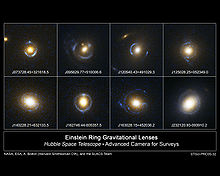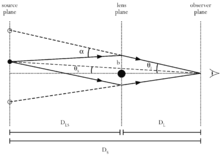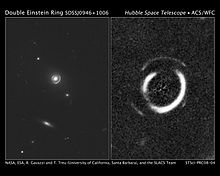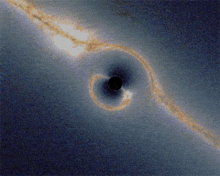# Einstein ring

Einstein ringSome observed Einstein rings

In observational astronomy an Einstein ring is the deformation of the light from a source (such as a galaxy or star) into a ring through gravitational lensing of the source's light by an object with an extremely large mass (such as another galaxy, or a black hole). This occurs when the source, lens and observer are all aligned. The first complete Einstein ring, designated B1938+666, was discovered by collaboration between astronomers at the University of Manchester and NASA's Hubble Space Telescope in 1998.

## Introduction

Gravitational lensing is predicted by Albert Einstein's theory of general relativity. Instead of light from a source traveling in a straight line (in three dimensions), it is bent by the presence of a massive body, which distorts spacetime. An Einstein Ring is a special case of gravitational lensing, caused by the exact alignment of the source, lens and observer. This results in a symmetry around the lens, causing a ring-like structure.The geometry of a gravitational lens

The size of an Einstein ring is given by the Einstein radius. In radians, it is$\theta_E = \sqrt{\frac{4GM}{c^2}\;\frac{d_{LS}}{d_L d_S}},$

where

G is the gravitational constant,
M is the mass of the lens,
c is the speed of light,
dL is the angular diameter distance to the lens,
dS is the angular diameter distance to the source, and
dLS is the angular diameter distance between the lens and the source.

Note that, over cosmological distances$d_{LS}\ne d_S-d_L$ in general.

## History

The bending of light by a gravitational body was predicted by Einstein in 1912, a few years before the publication of General Relativity in 1916 (see Renn et al. 1997). The ring effect was first mentioned in academic literature by Orest Chwolson in 1924. Albert Einstein remarked upon this effect in 1936 in a paper prompted by a letter by a Czech engineer, R W Mandl , but stated

Of course, there is no hope of observing this phenomenon directly. First, we shall scarcely ever approach closely enough to such a central line. Second, the angle β will defy the resolving power of our instruments.
Science vol 84 p 506 1936

In this statement, β is the Einstein Radius currently denoted by θE (see above). However, Einstein was only considering the chance of observing Einstein rings produced by stars, which is low; however, the chance of observing those produced by larger lenses such as galaxies or black holes is higher since the angular size of an Einstein ring increases with the mass of the lens.

### Known Einstein rings

Hundreds of gravitational lenses are currently known. About half a dozen of them are partial Einstein rings with diameters up to an arcsecond, although as either the mass distribution of the lenses is not perfectly axially symmetrical, or the source, lens and observer are not perfectly aligned, we have yet to see a perfect Einstein ring. Most rings have been discovered in the radio range.

FOR J0332-3557 03h:32m:59s:94, -35°57'51".7, J2000 1".48 Partial, 260° Radio Cabanac (2005)
SDSSJ0946+1006 09h 46m 56.s68, +10° 06' 52."6 J2000 Optical Gavazzi (2008)
MG1131 + 0456

## Extra ringsSDSSJ0946+1006 is a Double Einstein Ring. Credit: HST/NASA/ESA

Using the Hubble Space Telescope, a double ring has been found by Raphael Gavazzi of the STScI and Tommaso Treu of the University of California, Santa Barbara. This arises from the light from three galaxies at distances of 3, 6 and 11 billion light years. Such rings help in understanding the distribution of dark matter, dark energy, the nature of distant galaxies, and the curvature of the universe. The odds of finding such a double ring are 1 in 10,000. Sampling 50 suitable double rings would provide astronomers with a more accurate measurement of the dark matter content of the universe and the equation of state of the dark energy to within 10 percent precision.

### A simulationEinstein rings near a black hole

To the right is a simulation depicting a zoom on a Schwarzschild black hole in front of the Milky Way. The first Einstein ring corresponds to the most distorted region of the picture and is clearly depicted by the galactic disc. The zoom then reveals a series of 4 extra rings, increasingly thinner and closer to the black hole shadow. They are easily seen through the multiple images of the galactic disk. Odd rings correspond to points which are behind the black hole (from the observer point of view) and correspond here to the bright yellow region of the galactic disc (close to the galactic center), whereas even rings correspond to images of regions which are behind the observer, which appear bluer since the corresponding part of the galactic disk is dimmer here.

Wikimedia Foundation. 2010.

### Look at other dictionaries:

• Einstein-Ring — Bei einem Einsteinring handelt es sich um einen Ring elektromagnetischer Strahlung eines weit entfernten Objekts, der durch die Wirkung der Gravitation einer Vordergrund Galaxie zustande kommt. Die Galaxie wirkt dabei als Gravitationslinse.… …   Deutsch Wikipedia

• Einstein radius — The Einstein radius is the radius of an Einstein ring, and is a characteristic angle for gravitational lensing in general, as typical distances between images in gravitational lensing are of the order of the Einstein radius. Derivation In the… …   Wikipedia

• Einstein-Radius — Bei einem Einsteinring handelt es sich um einen Ring elektromagnetischer Strahlung eines weit entfernten Objekts, der durch die Wirkung der Gravitation einer Vordergrund Galaxie zustande kommt. Die Galaxie wirkt dabei als Gravitationslinse.… …   Deutsch Wikipedia

• Einstein Cross — Quasar name = QSO 2237+0305 epoch = J2000 ra = RA|22|40|31 dec = DEC|+3|21|30.3 constellation name = Pegasus z = 1.695 dist ly = 8,000,000,000 ly (2,500,000,000) pc type =LeQ appmag v =16.78 size v = 0.870´ X 0.338´ notes = names =LEDA 69457, Z… …   Wikipedia

• Einstein-Kreuz — Datenbanklinks zu Einsteinkreuz Galaxie Daten vom Einsteinkreuz …   Deutsch Wikipedia

• Ring singularity — In general relativity the gravitational singularity at the center of a rotating black hole (a Kerr black hole ) is supposed to form a circle rather than a point. This is often referred to as a ring singularity.Description of a ring… …   Wikipedia

• Ring (Stephen Baxter novel) — Infobox Book name = Ring title orig = translator = image caption = First edition cover author = Stephen Baxter cover artist = country = Great Britain language = English series = Xeelee Sequence genre = Science fiction novel publisher = Voyager… …   Wikipedia

• Anillo de Einstein — Saltar a navegación, búsqueda Anillos de Einstein vistos por el Hubble En astronomía de observación un anillo de Chwolson o anillo de Einstein es una deformación de la luz de una fuente lumínica (como una galaxia o una estrella) en anillo por …   Wikipedia Español

• List of things named after Albert Einstein — This is a list of things named after Albert Einstein. Scientific and mathematical concepts * Higher dimensional Einstein gravity * Einstein solid * Einstein force * Einstein s constant * Einstein relation (kinetic theory) * Stark Einstein law *… …   Wikipedia

• Lieserl Einstein — Infobox Person name = Lieserl Einstein image size = caption = birth name = Lieserl Einstein birth date = birth date|1902|2|4 birth place = Novi Sad death date = Unknown death cause = Unknown resting place = Unknown resting place coordinates =… …   Wikipedia